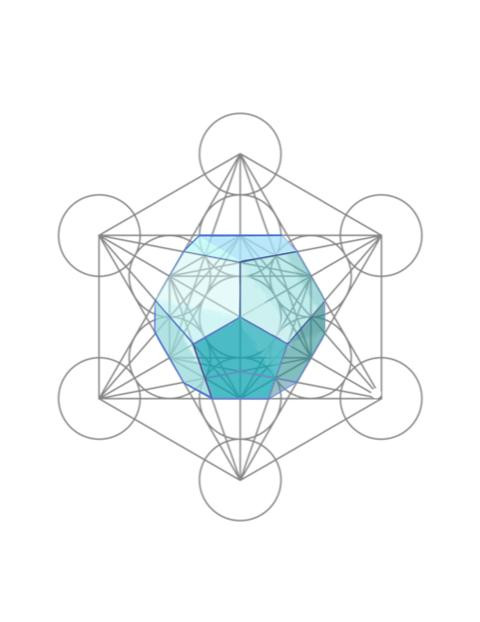Search

# Metatron's Cube - Hiding in Plain SightSo far we have discussed the first three platonic solids that are contained in Metatron's cube. They are the tetrahedron, the hexahedron, and the octahedron. I hope you are beginning to see how these fit together in three dimensional space. If that image isn't clear in your head, you might want to go back and review the previous two blogs making note of the pictures that are included in them.

In this blog I'm going to discuss the two remaining Platonic solids, the more complex shapes of the dodecahedron which is a twelve-sided polyhedron and the icosahedron which is a twenty-sided polyhedron. Each side or face of the dodecahedron is in the shape of a pentagon. So, there are five points or vertices to each one of the faces.

And while it may seem more complex than the other platonic solids, it is really not so hard to build a dodecahedron. You begin with one pentagon and lay it flat on a surface and then you connect a pentagon to each one of its sides. Then you take a second pentagon and lay it on a flat surface and again connect a pentagon to each one of its sides. And then you take the two pieces and fit them together and that creates a dodecahedron.The interesting thing about the dodecahedron is that there are not only two different dodecahedrons within Metatron’s cube, a smaller one and a larger one, but each dodecahedron can be drawn from two different perspectives. The perspective that is shown in the picture that I've included is the smaller dodecahedron from one of the two perspectives. I'm not going to include in this blog the other perspective just to keep things simple.Let's move on to the icosahedron which as I mentioned earlier is a twenty-sided polyhedron. Each face of the icosahedron is a triangle. I won't go into how to build one because it's a little more complicated than the dodecahedron. Again, there are two icosahedrons within Metatron's cube and the picture that I've shown here illustrates the larger icosahedron.

The icosahedron and the dodecahedron are duals. And if you can imagine one inside of the other each vertex of the one image is going to touch the center face of the other image. So, if we imagine the smaller dodecahedron inside of the larger icosahedron then each vertex of the dodecahedron touches the center of each face of the icosahedron. You may wonder how that's possible since the dodecahedron only has 12 faces but remember each face is a pentagon. And if you were to invert the two putting the icosahedron inside of the dodecahedron each vertex of the icosahedron would touch the center of each face of the dodecahedron.

As we move from the dodecahedron to the icosahedron, we get closer to a two-dimensional circle or a three-dimensional sphere. Remember the circle/sphere is not one of the Platonic solids. There are, however, thirteen circles in Metatron’s cube and they have a special purpose. Here is a quote from the team about the circles.

“The circles are the key for without the circles you have no point of reference.”

We will discuss the circles/spheres in greater detail in the next blog.

I haven’t mentioned the star tetrahedron or Merkaba, as I like to call it, which is also inside Metatron's cube. It is pretty easy to see. I’ll talk more about it in the next blog. There is also one more shape in Metatron’s cube that we will discuss in the next blog and that is the tesseract. Take a good look at Metatron’s cube and see if you can find where the tesseract is.

We have discussed all five of the Platonic solids in the last three blogs. The important thing to remember is that Metatron’s cube is at least three-dimensional. Also keep in mind that each of these Platonic solids moves. They move in relationship to each other. I'll be going into the movement of the solids in much more detail in upcoming blogs.

The movement of the Platonic solids in relationship to each other creates energy. And we can use that energy for a variety of purposes one of which is healing our physical bodies. I hope you will continue to journey with me as we discover more about the mysteries of Metatron’s cube together.